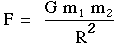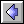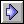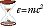### Newton's Laws

First, we must define our terms:

Speed is the distance traveled per time, or s = d / t.

Velocity is speed plus a direction.  In other words, "50 miles/hour" is a speed, "50 miles/hour going N by NW" is a velocity.

Acceleration is the change in the velocity per unit time, or:

a = [V (final) - V (initial)] / [t (final) - t (initial)]

Velocity has a direction, therefore acceleration has a direction.  For example, an object moving in a circle at constant speed is NOT moving at constant velocity, because the direction of its motion is constantly changing.  Therefore, its acceleration is also constantly changing, because the direction in which the object is being accelerated is changing.  In other words, it is not necessary for an object to be changing speeds in order to be accelerating.  In fact, it is not even necessary for the object to be moving!  For example, a rock thrown into the air comes momentarily to a stop when it reaches its maximum height.  It has zero velocity at this point.  But, it is STILL being accelerated downward at 9.8 m/s by the Earth's gravity, because its velocity is CHANGING from zero to not-zero, and it is the CHANGE in velocity that determines acceleration, not the velocity itself.  (Think about it -- if the acceleration was really zero for the rock at the top of its arc, then its velocity would not be changing.  And since its velocity at the top is zero, this means that its velocity would remain zero forever and the rock would just float there.)

You also need to realize that the above formulas for speed and acceleration actually give you the average speed or acceleration over the time interval in question.  For example, if you drive the 15 miles from your home to a friend's home in 30 minutes, then your average speed is 15 miles / 30 minutes = 0.5 miles per minute = 30 miles per hour.  However, unless you live in a very strange neighborhood, it is very unlikely that your speed was exactly 30 miles per hour for the entire journey.  Your instantaneous speed is your speed at a given instant, which in your car is conveniently measured by your speedometer.  Your instantaneous speed and your average speed become more and more nearly the same as you make the time interval over which you measure the speed smaller and smaller.

Newton's Laws of Motion are:

1)  All objects in motion in a straight line (or at rest) will remain in motion in a straight line (or remain at rest) unless acted upon by a force.
2)  F = ma
3)  For every action there is an equal and opposite reaction.
The equation F = ma actually defines what we mean by force.  (Note that force always has a direction.)  The mass of an object, m, which appears in this equation is simply a measure of how much matter the object contains.  The most intuitive way to think about mass is in terms of weight:  if you double the mass of an object, you will double its weight at the Earth's surface.

HOWEVER, it is critical to realize that mass and weight are not the same, and in fact, don't have anything specific to do with each other.  Mass is something that an object has, and is intrinsic to it.  Weight is merely the result of gravity pulling on the mass.  Astronauts on the Moon weigh about 1/6 of what they do on the Earth, because the Moon is much smaller than the Earth and so has weaker gravity.  However, the mass of the astronauts has not changed.  The mass of an object never changes, whereas the weight can be almost anything, depending on where the object is.  We can directly compare mass to weight on the Earth's surface (for example, on a bathroom scale) only because the gravity is more-or-less constant everywhere near the surface of the Earth.The momentum PR of the rifle must be equal and opposite to the momentum PB of the bullet, or mRvR = mBvB.
Newton's Third Law states that for every action there is an equal and opposite reaction.  An alternative way to think about this law is to say that forces always come in pairs:  each pulling with equal strength in opposite directions.

Examples of the Third Law:  If you jump off a small boat to the shore, the boat will move in the opposite direction from your jump.  Whenever you fire a gun, there is a strong "kick" backwards as the bullet shoots forward.  In situations where there may not seem to be any reaction, for example, a book sitting on a table, you have to remember that the table is pushing up on the book even as gravity pulls it down.  If you imagine the table being replaced by something more flexible, such as a light sheet of plastic, then you know the plastic will bend downward as more weight is placed on it.  If the weight is suddenly removed, then the plastic will snap back, demonstrating that it was exerting an upward force while it was bent.  A wooden table works exactly the same way, only much more stiffly.  Finally, when I walk forward and thus accelerate myself, there may not seem to be a reaction -- but as I walk forward I also push backwards on the Earth and accelerate it *very* slightly in the opposite direction.

NOTE -- The Second Law should really be written as F(net) = ma, because if you have more than one outside force acting -- say, two crews of people playing tug-of-war -- then the acceleration of the rope will be given by the vector sum of the forces on the rope, i.e., the net force.  For our tug of war, it may well be possible that the rope is not moving at all and has no acceleration, but this only means that there is no NET force acting on the rope.  There are still forces present, which you can easily discern if the teams pull too hard and break the rope.

A few comments about the confusion caused by mixing metric and English units.  In the metric system, the kilogram is a unit of MASS (I cannot emphasize this too strongly), whereas the unit of force is called a newton.  Mass and force have nothing to do with each other, except through the equation F = ma.

In the English system, however, pounds are a unit of FORCE -- which leads to endless confusion since junior-high-school teachers everywhere in America keep telling their students that one kilogram "equals" 2.2 pounds.  (Or, you can go the grocery store and buy a pound of cookies, where the label implies that one pound is "equal" to 454 grams.)

Wrong.  A pound is a force and a kilogram is a mass and equating them is like comparing apples and oranges.

The deal is, the acceleration due to gravity at the Earth's surface is equal to 9.8 m/s2.  So, if you put a one kilogram mass onto a metric scale and weigh it, the scale will say 9.8 newtons, because the newton is the metric unit of force.  If the same kilogram mass is put onto an English scale, then the scale will measure the force in English units and say 2.2 pounds.  In other words, a one-kilogram mass exerts 2.2 pounds of gravitational force -- if you happen to be on the surface of the Earth.  If you are anywhere else, this is not true.  The gravity will be different and thus the force exerted (in pounds or newtons) will change.  In deep space, for example, there is no gravity so a = 0 which means F = ma = 0.  So, according to our English scale, it takes an infinite number of kilograms in space to equal one pound, because no matter how many you pile on, they still weigh nothing.  The mass of our kilogram weight, on the other hand, remains constant regardless of gravity, because mass tells you how much "stuff" is there.

So, if you are always on the surface of the Earth, where the gravity is always more-or-less the same, then (and only then) can you make the statement that a kilogram is "equal" to 2.2 pounds.  This is OK for selling cookies, but it is just confusing if you are talking basic physics.  It is good to remember that the conversion of 2.2 lbs (English weight of kilogram)= 9.8 newtons (metric weight of kilogram), or one lb = 4.45 N, is always true, because now you are comparing forces to forces.  You could do the same thing by comparing masses to masses, but the unit of mass in the English system is a thing called a slug, which is so obscure you could probably use it as a question on Who Wants To Be A Millionaire.  (One slug is the amount of mass that would weigh 32 lbs on the Earth's surface, if you are wondering.)

Back to physics.  The Law of Universal Gravitation states that all objects in the universe attract each other with a force given by:, where:
• m1 and m2 are the masses of the objects
• G is the gravitational constant (6.7 X 10-11 in metric units)
• and r is the distance separating the two masses.
In the Principia, Newton proved that the planets must obey Kepler's Laws if universal gravitation is correct.  Even better, he was able to explain the slight deviations from Kepler's Laws which Jupiter and Saturn exhibit, by showing that they gravitationally attract each other.  Kepler never knew it, but mathematically his laws are strictly correct only if you have two bodies interacting.  Kepler hadn't noticed because the Sun is so overwhelmingly more massive than the planets that, to a good approximation, each planet just orbits in the Sun's gravitational field almost independently of the other planets.  This is pretty close to a perfect two-body system.  But in the case of Jupiter and Saturn, the two most massive planets, they do tug on each other enough to make their orbits deviate slightly from Kepler's Laws when they are close together.  This had been noticed by telescopic astronomers after Kepler's death, but never explained until Newton.

Newton also predicted that a pendulum would swing more slowly at the equator than in England (due to the centrifugal force of the Earth's rotation).  He correctly explained the tides as resulting from gravitational interaction between the Earth and Moon.  He was able to show that the precession of the Earth's axis of rotation (the so-called precession of the equinoxes) is due to the fact that gravitational forces from the (apparent) rotations of the Sun and Moon around the Earth place a twisting force on the Earth's equatorial bulge.

Newton's physics can be thought of as the first "correct" physics, in the sense that it is still taught today and universally used by modern physicists and engineers.  We pay suitable homage to the ancient Greeks for their ground-breaking belief that the Universe is governed by logical, mathematical laws rather than by Gods, and to their early attempts to rationally explain the Universe.  We recognize the impressive accomplishments of men such as Copernicus and Kepler.  But alas, in the end, most of their ideas have proven to be wrong or at best only rough approximations.  (Galileo is perhaps the one shining exception.)  The physics of the 21st century begins with Isaac Newton.Newton page  |  Energy pageIdeas of Physics Homepage